# Detailed support vector machine

Posted Jun 15, 202010 min read

Author|Anuj Shrivastav
Compile|VK
Source|Medium### Introduction

Supervised learning describes a type of problem that involves using models to learn the mapping between input examples and target variables. If there is a classification problem, the target variable can be a class label, and if there is a regression problem, the target variable is a continuous value. Some models can be used for regression and classification. One such model we will discuss in this blog is the support vector machine, or SVM for short. My purpose is to provide you with simple and clear internal work of SVM.

Suppose we are dealing with binary classification tasks.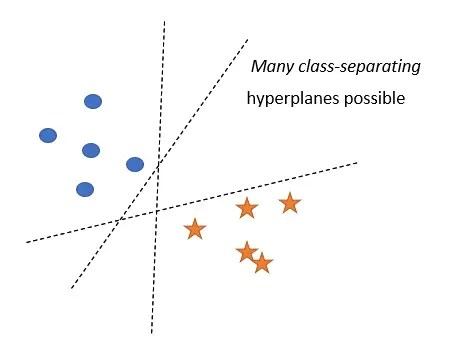There may be an infinite number of hyperplanes that can separate these two classes. You can choose any of them. But can this hyperplane predict the class of new query points well? Don't you think the plane close to one class is good for another class? Intuitively, the best way to separate the two classes is to choose a hyperplane that is equidistant from the closest point in the two classes.

This is the role of SVM!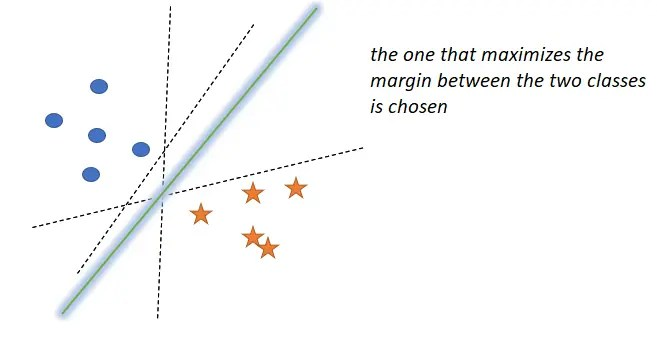The core idea of support vector machine is:

Choose the hyperplane that separates the +ve point and the -ve point as widely as possible.(ve stands for vector, vector, which is the vector of samples)

Let be the hyperplane separating these two types, + and _ are the two hyperplanes parallel to

is the plane we get when we move parallel to and touch the nearest +ve point of

is the plane we get when we move parallel to and touch the nearest-ve point of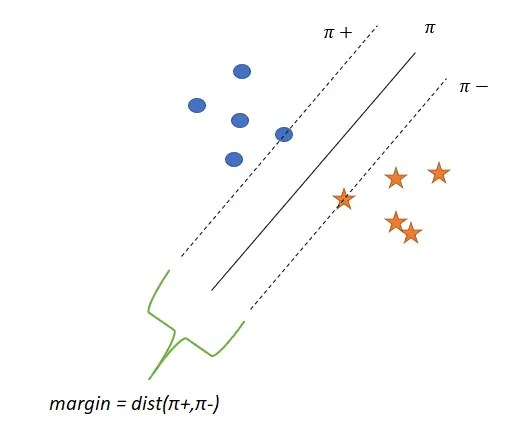d=margin = dist( , )

• SVM tries to find a to maximize the interval.
• As the interval increases, the generalization accuracy also increases.

#### Support Vector

Points on or are called support vectors.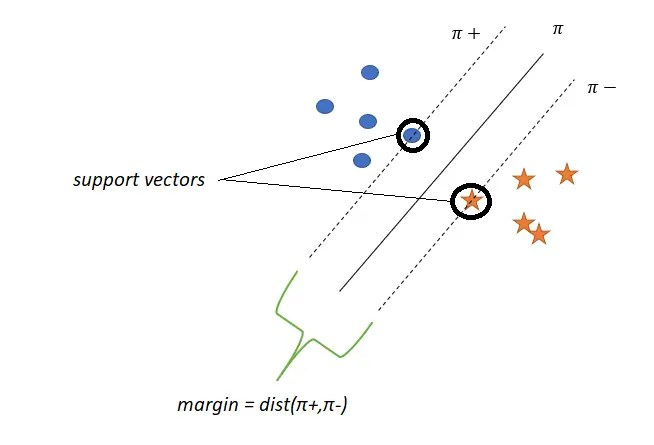### SVM mathematical formula

:hyperplane with maximum margins

:w x+b=0

Assume

:w x+b=+1

Any point on or any point away from in the positive direction is marked as positive

:w x+b=-1

Any point on or any point away from in the negative direction is marked as negative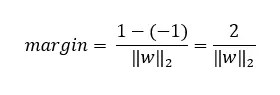The optimization problem is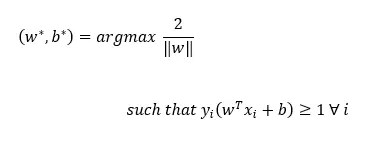Now, this looks good, but it only works when our data is linearly separable. Otherwise, we will not be able to solve the above optimization problem, nor will we be able to obtain optimal w and b.

Suppose a scenario where the data is not linearly separable:Because of these four points, our optimization problem will never be solved, because these points y(w x+b) are not greater than 1.

This is because our optimization problem is too strict, it only solves linearly separable data. Hard margin is the name of this method.

So, can we modify it? Can we be slightly wider so that it can handle almost linearly separable data?What we have to do is, we make a relaxation variable (zeta), corresponding to each data point, so that for the +ve point in the +ve region and the -ve point in the -ve region,

=0.

This leaves us with wrong classification points and points within the interval.

The meaning of , take the picture as an example, when =2.5

This means that pt.4 is 2.5 units away from its correct hyperplane( in this case) in the opposite direction.

Similarly, pt.1 is opposite to the direction of the optimal hyperplane( in this example), with a distance of 2.5 units.

As increases, the point is further away from the optimal hyperplane in the wrong direction.Improve optimization problemsLet's break it down

First, the constraints:

we know,right now:withc is the hyperparameter.

We can intuitively think of the optimization problem as:If the value of c is higher, the weight of the loss term is greater, resulting in overfitting of the data.

If the value of c is low, the weight of the regularization term is large, resulting in under-fitting of the data.

#### SVM dual form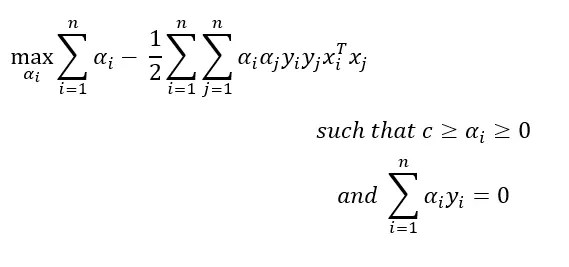Wait! How do we get this dual form?

It's all about optimization. If we study this issue in depth, we will deviate from our goal. Let's discuss this dual form in another blog.

Why do we need this dual form?

The dual form is sometimes easier to solve. If the dual gap is very small, we will get similar results. Support vector machine in particular:the dual form is very important because it opens up a new way of explaining support vector machines through kernel functions(I will tell you later in this blog).

Note:In the dual form, all x appears as a dot product, unlike the original form where all x appears as independent points.

can be considered as a Lagrange multiplier

Observe the dual form:

• For each x , there is a corresponding
• All x are in the form of dot products
• Our classification of a new point is changed as follows: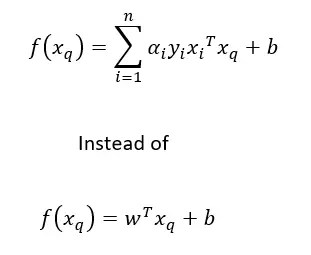• Support vector >0, non-support vector =0.

This means that only support vectors are important, which is why the model is named a support vector machine.

Now, in dual form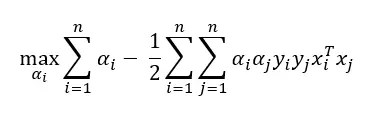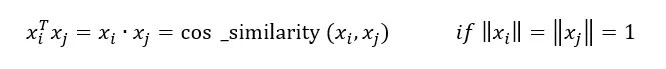Therefore, if a similarity matrix is given, we can use the dual form instead of the original form. This is the advantage of support vector machines. Generally, this similarity(x , x) is replaced by K(x , x), where K is called a kernel function.

#### Nuclear skills and the intuition behind them

Replacing the similarity(x , x) with the kernel function K(x , x) is called kernelization, or applying nuclear techniques.

If you look at it, it just calculates the dot product of x and x . So, what's so great?

Let us take the following data set as an example.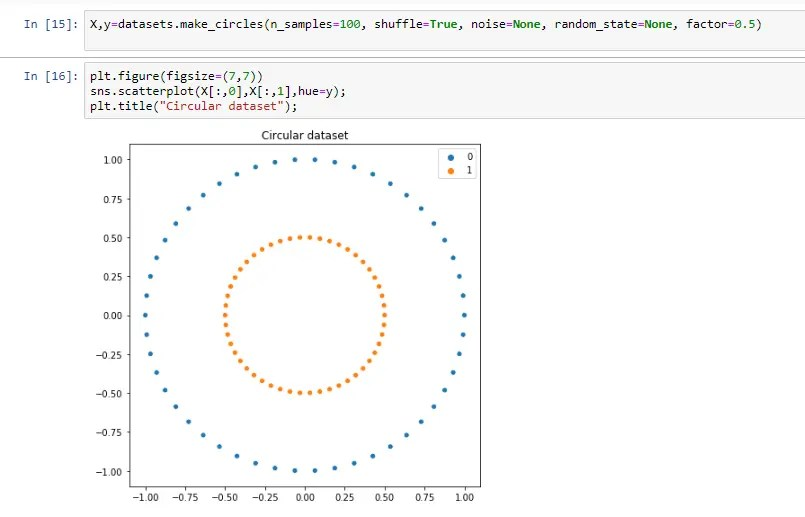Obviously, with the help of linear models, these two classes cannot be separated.

Now, convert the dataset to a cube with the characteristics of <x ²,x ²,2x x >.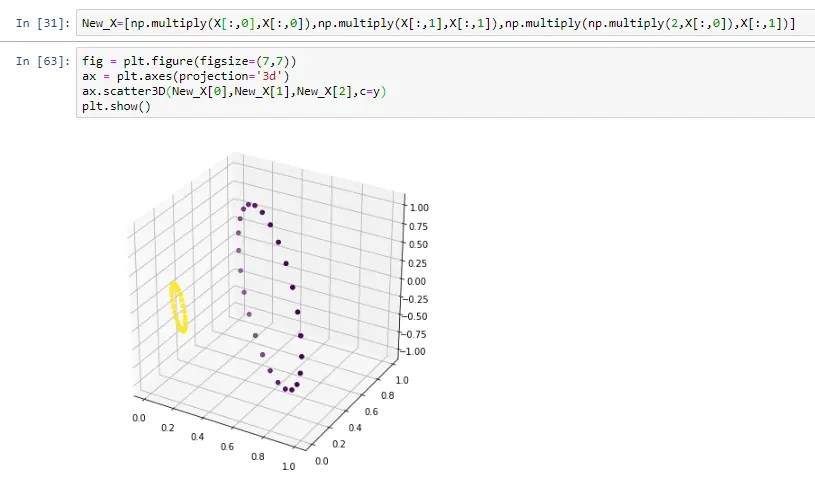Did you see it? Apply proper feature transformation and increase the dimension to make the data linearly separable.

This is the role of nuclear support vector machines. It maps the original features to a high-dimensional space, finds the hyperplane with the largest interval in the space, and maps the hyperplane to the original dimensional space to obtain a nonlinear decision surface without actually accessing the high-dimensional space .

and so

Linear support vector machine:find the hyperplane with maximum interval in x space

Kernel support vector machine:find the hyperplane with maximum interval in the transformation space of x

Therefore, the kernel support vector machine can also solve nonlinear separable data sets.

Let's look at some kernel functions used in support vector machines-

#### Polynomial kernel

The definition is as follows: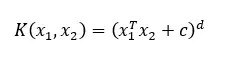among them

d=Data dimension

c=constant

For the secondary kernel, set c=1, that isWhen the dimension is 2This can be thought of as the product of 2 vectors x and x , where:Now dimension=d'=6

Therefore, nucleation and feature conversion are the same, but usually d'>d, nucleation is done implicitly internally.

It is the most popular core, because you can t decide which core to choose, you can choose it

The definition is as follows:The effect of d:

As d increases, the numerator of the exponential part decreases, in other words, the K value or similarity decreases.

The effect of :If you pay attention, when =0.3, at x=2, it is close to 0. At x = 4, = 1 and x = 11, = 10, it is close to 0. This shows that when increases, even if the two points are far away, the similar score may not be very low.

For example, suppose that there are two points x and x with a distance of 4 units. If we apply RBF kernel with =0.3, the kernel function K value or similar value is 0. If we set =1, the K value is very close to 0, but if we set =10, the K value is about 0.4.

Now explain the intuition behind the RBF core

Remember the 6-dimensional mapping function we got when using the polynomial kernel function? Now let us try to find a mapping function of the RBF kernel.

To simplify the math, suppose the original data has a dimension of 2, and the exponent part has a denominator of 1. under these circumstances,If we try to find the mapping function of the RBF kernel, we will get an infinite vector. This means that the RBF kernel requires our data to be mapped into an infinite dimensional space, calculate the similarity score and return it, which is why if you don't know which kernel to choose, the RBF kernel function will be the safest choice.

### SVM for regression

So far, we have studied how to perform classification tasks using support vector machines. But support vector machines are not limited to this. It can also be used to perform regression tasks. how? let us see!

First, let us look at the mathematical formula of support vector regression(SVR)The way SVR works is that it tries to find a hyperplane with the most suitable data points while maintaining a soft interval = (hyperparameter), which means that all points should be distance on both sides of the hyperplane.

Minimizing the boundary here means that we want to find a hyperplane that matches the data with a low error.

Note:The square is to make the function differentiable and suitable for optimization. The same can be done for SVC.

What's wrong with this formula?

The problem here is that we are too strict and the distance that we expect the point to be in the hyperplane does not happen often in the real world. In this case, we will not be able to find the required hyperplane. So, what should we do?

In the same way as we deal with this problem in SVC, we will introduce two relaxation variables and * for normal points to allow certain points to be outside the range, but will be punished.So the mathematical formula now becomes:Where C determines the required rigor. The larger the value of C, the greater the penalty for points outside the margin, which may lead to overfitting of the data. Fewer C means less penalties for points beyond the edge, which may result in insufficient data fitting.

As in SVC, the diagram shows the original form of SVR. The results show that the dual form is easier to solve, and the nonlinear hyperplane can be obtained by using kernel techniques.

As I have said, the formula in the dual form is a bit tricky and involves knowledge of solving constrained optimization problems. We will not discuss too many details in depth, because this will divert our attention to support vector machines.

#### SVR dual form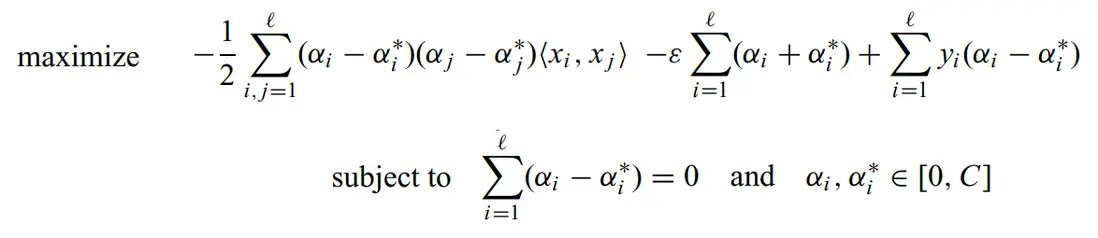This is obtained by using Lagrange multipliers to solve the optimization problem.

For a new point, our method of calculating the output value is: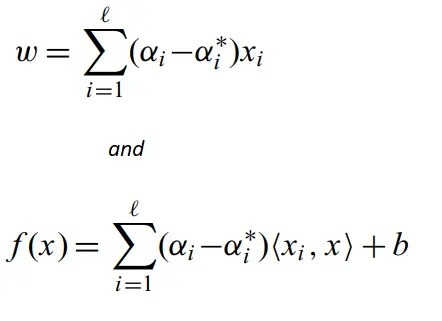Have you noticed that x is in the form of a dot product? Yes, it is the same as what we got in SVC. We can replace the dot product with the previously mentioned similarity or kernel function. Applying nuclear techniques can also help us fit nonlinear data.

### Various situations of support vector machine

1. Feature engineering and feature conversion, which is achieved by finding the correct kernel discussed above
2. Decision surface, for linear support vector machine:the decision surface is just a hyperplane, for kernel support vector machine:it will be a nonlinear surface
3. Similar function/distance function, the original form of support vector machine cannot handle similar function. However, due to the existence form of x dot product, the dual form can easily handle it.
4. Importance of features. If the features are not collinear, the weight of the features in the weight vector w determines the importance of the features. If the features are collinear, you can use forward feature selection or backward feature elimination, which is the standard method for determining the importance of any model feature.
5. Outliers, compared with other models such as Logistic regression, support vector machines have less impact.
6. Deviation-variance, which depends on the value of c in the dual form of the support vector machine.

If the value of c is high, the weight of the error term is large, so the model may overfit the data; if the value of c is low, the weight of the error term is small, and the model may underfit the data.

1. High dimension, the design of support vector machine can work well even in high dimension. As shown in the figure, there is already a regularization term in the mathematical formula of the support vector machine, which helps to deal with high-dimensional problems. You might say that other models like KNN are not suitable for high-dimensional spaces, so what's so special about SVMs? This is because support vector machines only care about finding the plane that maximizes the margins, and not between the points. relative distance.

### Advantages of support vector machines

• It has a regularization term to help avoid overfitting of data
• It uses nuclear techniques, which helps to process even non-linear data(in the case of SVR) and non-linear separable data(in the case of SVC)
• When we don't understand the data, support vector machine is very good.
• Can deal with unstructured and semi-structured data, such as text, images and trees.
• In a sense, it is robust and works even if the training example contains errors

### Limitations of support vector machines

• Choosing the right core is a difficult task.
• The support vector machine algorithm has multiple hyperparameters that need to be set correctly to obtain the best classification results for any given problem. The parameters may cause the classification accuracy of problem A to be very good, but may cause the classification accuracy of problem B to be very poor.
• When the number of data points of the support vector machine is large, the training time is long.
• For kernel support vector machines, the analysis of the weight vector w is more difficult.